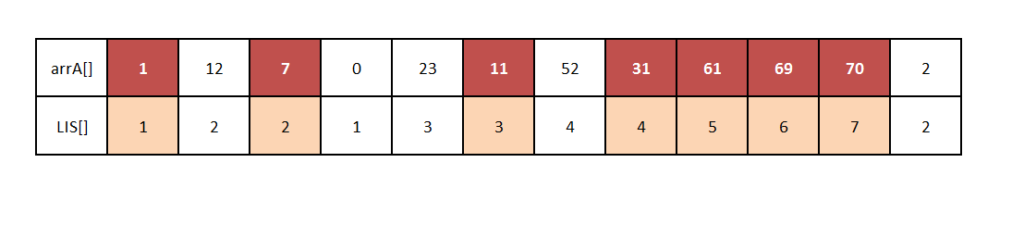# Length of Longest Increasing Subsequence in Java

In this Java tutorial, we are going to discuss how to find the length of longest increasing subsequence in an array. Longest increasing Subsequence problem is being asked in competitive programming problem many times and is also important from interview point of view.## What does Arrays.sort() do in Java?

Arrays.sort() function sort the array in ascending order . Header file needed to use this function is

import java.util.Arrays;

Syntax : – Arrays.sort ( arr ) ;

where arr is the array which is needed to be sort.

### Code Snippet to update array l[]

```for(i=0;i<n;i++)
{
for(j=0;j<i;j++)
{
if(a[i]>=a[j])

l[i]=Math.max(l[j]+1,l[i]);
}
}```

### Algorithm

1. Take two array a[] and l[] where a[] is your original array and l[] array keeps the length of increasing subsequence for each element.
2. Initialize l[] with value 1 because every element will also be considered in length of increasing subsequence.
3. Update the value of l[i] for every ith element in array a[] by keeping track of how many elements are smaller than ith element from index 0 to i-1.
4. After updating array l[] for each element , find maximum value in array l[] by sorting array l[] using Arrays.sort() and return l[n-1].

### Java Code to print length of longest increasing subsequence

```import java.util.Scanner;

import java.util.Arrays;

public class Codespeedy

{
public static int longest_increasing_subsequence_length(int a[] , int l[] , int n)
{
int i,j;

for(i=0;i<n;i++)
{
for(j=0;j<i;j++)
{
if(a[i]>=a[j])

l[i]=Math.max(l[j]+1,l[i]);
}
}

Arrays.sort(l);

return l[n-1];
}

public static void main(String[] args)

{
Scanner scan = new Scanner (System.in);

int n = scan.nextInt();

int a[] = new int[n];

int l[] = new int[n];

int i;

for(i=0;i<n;i++)

{
a[i] = scan.nextInt();

l[i]=1;
}

System.out.println(longest_increasing_subsequence_length(a,l,n));
}

}```

INPUT

```8
6 2 5 1 7 4 8 3```

OUTPUT

`4`

In the above example, the longest increasing subsequence is [ 2 , 5 , 7 ,8]. So, the length of the longest increasing subsequence is 4.